MODULE V Electrical engineering - Basics Page 19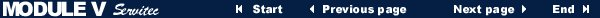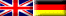3.2 Electric power

 Measurement unit W Watt Measurement unit N m Newton-Meter (Sir Isaac Newton, English Physicist, 1642-1727) Unit symbol P Power

If only ohmic resistors are present in direct current and alternating current circuits, then the electric power depends on the applied electric voltage and amperage.

In the case of alternating current and three-phase motors one has to additionally consider that the theoretical electric power, the so called "apparent power", is being reduced by capacitive resistors (e.g. condensors) or inductive resistors (e.g. coils). This power reduction is expressed by the factor cos j ("Cosinus Phi"). Cos j ranges from 0,5 to 0,9 depending on the form and quality of the considered electric part. The electric power that has been adjusted by the factor cos j is also called "active power".
Another factor, the so called "concatenation factor" (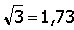), considers the concatenation and overlapping of the three outer conductors L1, L2 and L3 in the case of three-phase motors.

The following formula is used to calculate the electric power:

Electric power = Voltage * Amperage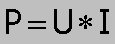or: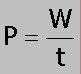Taking the discussed power reducing factors into consideration the formula can be extended in the following way:

Output power ("active power") for alternating current motors: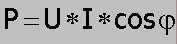Output power ("active power") for three-phase motors: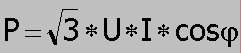• • Copyright © IWB e.V. 1996-2000 • Design, technical realisation and webmaster: ohlendorf@ohlendorf.de • •
• • Sponsored by EDUVINET • 800 x 600 recommended • •